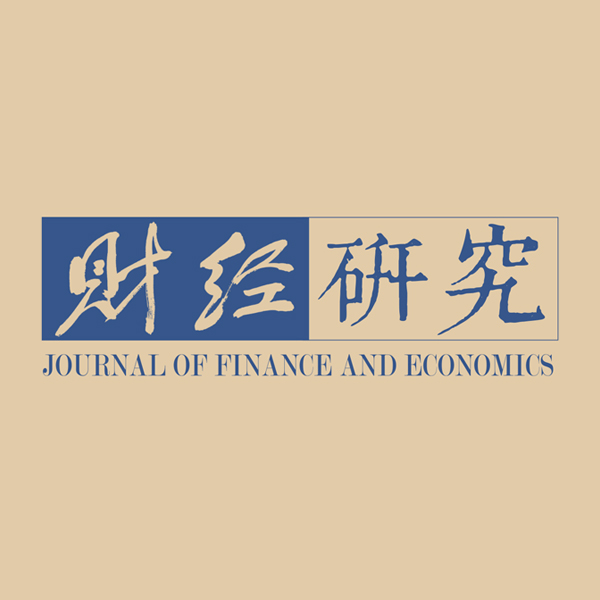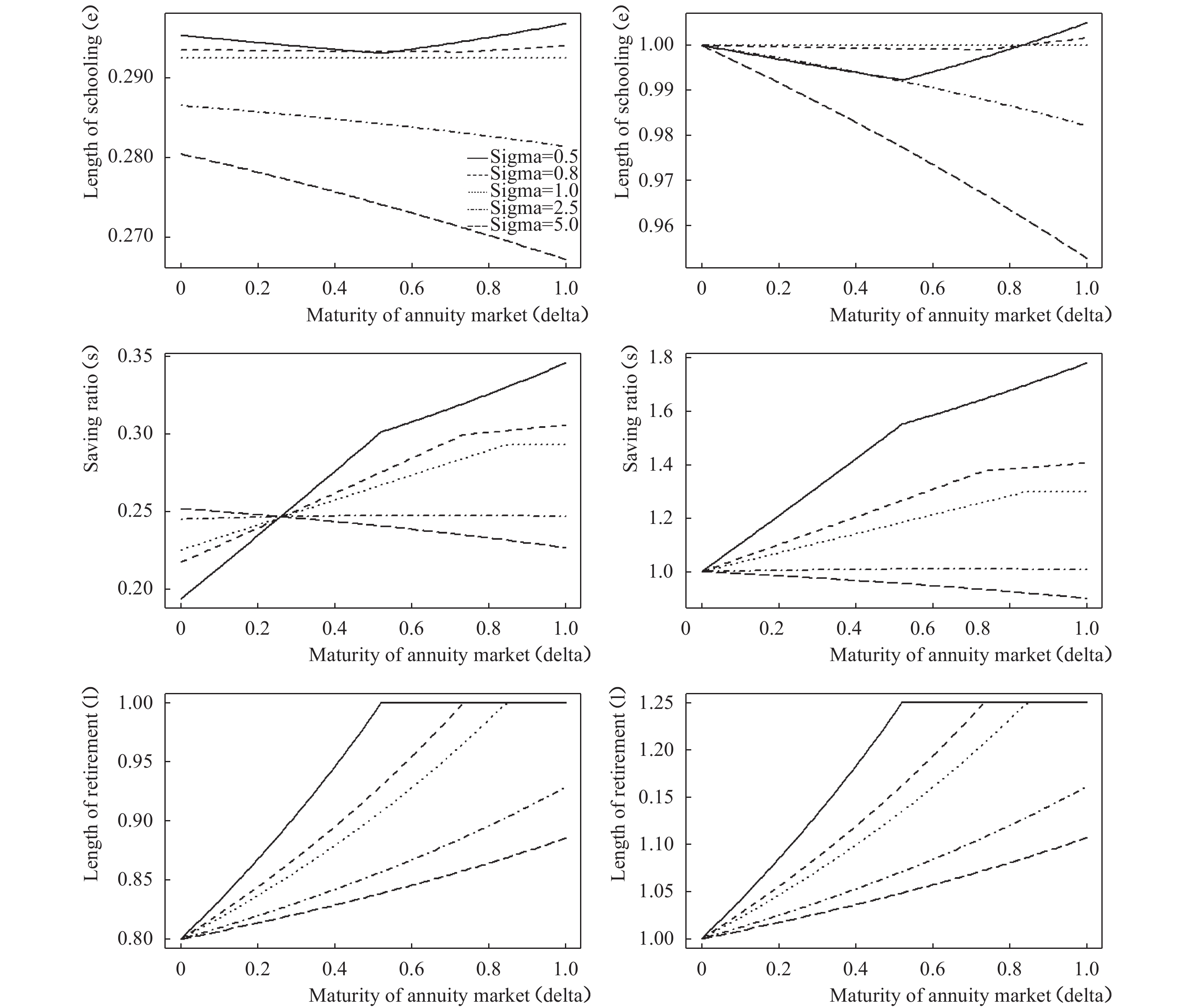﻿ 预期寿命、年金市场与经济行为《财经研究》
2019第45卷第2期

Life Expectancy, Annuity Markets and Economic Behaviors
Liu Pan, Wang Xinyu
Summary: In the past century, life expectancy in most countries has increased. At the same time, there is an increasing demand for the development of annuity markets. In this paper, we construct a three-period life-cycle model with credit constraints and annuity markets, and investigate the effects of increasing life expectancy and the development of annuity markets on individuals’optimal choices of the length of schooling, saving behavior, retirement length and expected lifetime total labor supply. 　　Within this framework, we find that as life expectancy increases, individuals choose to gain more education and increase savings, but how they are going to change the retirement decision and expected lifetime labor supply depends on the maturity of annuity markets and the interest rate in the economy. If there is no mature annuity market, or if the interest rate is low, as life expectancy increases, individuals will retire earlier. However, since the survival rate also increases, expected lifetime labor supply may not decline even when labor supply at the two end stages of life does. We also find that if life expectancy remains unchanged, as annuity markets develop, individuals will retire earlier. The robustness of the results has been checked with numerical examples.　　Our work is part of an important literature that tries to explain the" Ben-Porath puzzle”raised by Hazan（2009）, who shows that a necessary condition for the Ben-Porath mechanism（Ben-Porath, 1967）to hold is that increased life expectancy must also increase expected lifetime labor supply, which is not supported by empirical evidence. The main contributions of our paper are as follows: First, we introduce the annuity market, which is closely linked with life expectancy and retirement, to the model when revisiting the Ben-Porath mechanism. We emphasize the importance of annuity markets when analyzing the effects of increasing life expectancy on individuals’behaviors, and also show that the development of annuity markets will have an effect on individuals’optimal choice. Second, we not only analyze the effects of increasing life expectancy and the development of annuity markets on individuals’retirement decision, but also focus on the effects on individuals’expected lifetime labor supply, which is affected by both mortality rates and labor supply in each period. This makes our results comparable to the empirical findings by Hazan（2009）.
Key words: life expectancy    annuity markets    education attainment    savings    retirement decision

 ${c_1} = wh\left( e \right)\left( {1 - e} \right)$ (1)

 ${c_2} = wh\left( e \right) - S$ (2)

 ${c_3} = wh\left( e \right)\left( {1 - l} \right) + S\tilde R$ (3)

 $U = \ln{c_1} + \beta \ln{c_2} + \varphi {\beta ^2}\left( {\ln{c_3} + \theta \ln l} \right)$ (4)

 ${\cal L} = \ln\left[ {wh\left( e \right)\left( {1 - e} \right)} \right] + \beta \ln\left[ {wh\left( e \right) - S} \right] + \varphi {\beta ^2}\left\{ {\ln\left[ {wh\left( e \right)\left( {1 - l} \right) + S\tilde R} \right] + \theta \ln l} \right\} + \lambda \left( {1 - l} \right).$

Kuhn-Tucker条件为：

 ${e:\frac{{h'\left( e \right)}}{{h\left( e \right)}} - \frac{1}{{1 - e}} + \beta \frac{{wh'\left( e \right)}}{{wh\left( e \right) - S}} + \varphi {\beta ^2}\frac{{wh'\left( e \right)\left( {1 - l} \right)}}{{wh\left( e \right)\left( {1 - l} \right) + S\tilde R}} = 0}$ (5)
 $l:\varphi {\beta ^2}\left[ {\frac{{ - wh\left( e \right)}}{{wh\left( e \right)\left( {1 - l} \right) + S\tilde R}} + \theta \frac{1}{l}} \right] - \lambda = 0$ (6)
 $S:\beta \frac{{ - 1}}{{wh\left( e \right) - S}} + \varphi {\beta ^2}\frac{{\tilde R}}{{wh\left( e \right)\left( {1 - l} \right) + S\tilde R}} = 0$ (7)
 ${\lambda :1 - l {\text{≥}} 0,\lambda {\text{≥}} 0;\lambda \left( {1 - l} \right) = 0}$ (8)

 内点解 ${\left( {\tilde R \leqslant \displaystyle\frac{{1 + \varphi \beta }}{{\varphi \beta \theta }}} \right)}$ 端点解 ${\left( {\tilde R > \displaystyle\frac{{1 + \varphi \beta }}{{\varphi \beta \theta }}} \right)}$ 青年期接受教育年限（e） ${\left( {1 + \beta + \varphi {\beta ^2}} \right)\displaystyle\frac{{h'\left( e \right)}}{{h\left( e \right)}} - \displaystyle\frac{1}{{1 - e}} = 0}$ 中年期储蓄率 ${\left( {s = \displaystyle\frac{S}{{wh\left( e \right)}}} \right)}$ ${{s_1} = \displaystyle\frac{{\tilde R\varphi \beta \left( {1 + \theta } \right) - 1}}{{\tilde R\left( {1 + \varphi \beta + \theta \varphi \beta } \right)}}}$ ${{s_2} = \displaystyle\frac{{\varphi \beta }}{{1 + \varphi \beta }}}$ 老年期退休时长（l） ${{l_1} = \displaystyle\frac{{\theta \varphi \beta \left( {1 + \tilde R} \right)}}{{1 + \varphi \beta + \theta \varphi \beta }}}$ l2=1 青年期消费（c1） ${c_1^1 = c_1^2 = wh\left( e \right)\left( {1 - e} \right)}$ 中年期消费c2 ${{{c_2^1} = \displaystyle\frac{{\tilde R + 1}}{{\tilde R\left( {1 + \varphi \beta + \theta \varphi \beta } \right)}}}wh\left(e\right)}$ ${{c_2^2 = \displaystyle\frac{1}{{1 + \varphi \beta }}wh\left( e \right)}}$ 老年期消费（c3） ${c_3^1 = \displaystyle\frac{{\varphi \beta \left( {\tilde R + 1} \right)}}{{1 + \varphi \beta + \theta \varphi \beta }}wh\left( e \right)}$ ${c_3^2 = \displaystyle\frac{{\varphi \beta \tilde R}}{{1 + \varphi \beta }}wh\left( e \right)}$

（一）年金市场对消费者行为的直接影响

 ${s_1} = \frac{1}{{1 + \varphi \beta + \theta \varphi \beta }}\left[ {\varphi \beta \left( {1 + \theta } \right) - \frac{1}{{\tilde R}}} \right]$

 $\frac{{\partial \tilde R}}{{\partial \varphi }} = - \frac{{\delta R}}{{{{\left( {1 - \delta + \delta \varphi } \right)}^2}}} = - \frac{{\delta \tilde R}}{{1 - \delta + \delta \varphi }} < 0$ (9)

 $\frac{\partial }{\delta }\left| {\frac{{\partial \tilde R}}{{\partial \varphi }}} \right| = R\frac{{1 + \delta - \delta \varphi }}{{{{\left( {1 - \delta + \delta \varphi } \right)}^3}}} > 0$ (10)

（二）预期寿命对接受教育年限的影响

 $\frac{{de}}{{d\varphi }} = - \frac{{{\beta ^2}\displaystyle\frac{{h'\left( e \right)}}{{h\left( e \right)}}}}{{\left( {1 + \beta + \varphi {\beta ^2}} \right)\displaystyle\frac{{h''\left( e \right)h\left( e \right) - {{\left[ {h'\left( e \right)} \right]}^2}}}{{{{\left[ {h\left( e \right)} \right]}^2}}} -\displaystyle \frac{1}{{{{\left( {1 - e} \right)}^2}}}}} > 0$

（三）预期寿命增加对储蓄率的影响

 $\frac{{ds}}{{d\varphi }} = \frac{{\partial {\rm{s}}}}{{\partial \varphi }} + \frac{{\partial {\rm{s}}}}{{\partial \tilde R}}\frac{{\partial \tilde R}}{{\partial \varphi }}$

1. 消费者在老年期先工作再退休（内点解）。由表1中储蓄率的表达式可计算：

 $\begin{array}{l} \displaystyle\frac{{\partial {s_1}}}{{\partial \varphi }} = \displaystyle\frac{{\beta \left( {1 + \theta } \right)\left( {\tilde R + 1} \right)}}{{\tilde R{{\left( {1 + \varphi \beta + \theta \varphi \beta } \right)}^2}}} > 0\\ \displaystyle\frac{{\partial {s_1}}}{{\partial \tilde R}} = \displaystyle\frac{1}{{{{\tilde R}^2}\left( {1 + \varphi \beta + \theta \varphi \beta } \right)}} > 0 \end{array}$

 $\frac{{d{s_1}}}{{d\varphi }} = \frac{{\partial {s_1}}}{{\partial \varphi }} + \frac{{\partial {s_1}}}{{\partial \tilde R}}\frac{{\partial \tilde R}}{{\partial \varphi }} > 0$ (11)

 $\frac{\partial }{{\partial \delta }}\left( {\frac{{d{s_1}}}{{d\varphi }}} \right) = \frac{{ - \beta \left( {1 + \theta } \right) - 1}}{{R{{\left( {1 + \varphi \beta + \theta \varphi \beta } \right)}^2}}} < 0$ (12)

2. 消费者在老年期完全退休（端点解）。如果消费者在老年期没有劳动供给，当假设对数效用函数时，实际利率变化引起的收入效应和替代效应互相抵消，不影响消费者储蓄行为。消费者储蓄行为的改变全部来自于正向效应：

 $\frac{{d{s_2}}}{{d\varphi }} = \frac{\beta }{{{{\left( {1 + \varphi \beta } \right)}^2}}} > 0$

（四）预期寿命增加对退休决策的影响

 $\mathop {\rm Max }\limits_{{c_3},l} \left( {\ln{c_3} + \theta \ln l} \right)$
 $s.t.{c_3} = wh\left( e \right)\left( {1 - l} \right) + {W^{old}}$

 ${w^{old}} \equiv \frac{{{W^{old}}}}{{wh\left( e \right)}} = {s_1}\tilde R$

 $\frac{{d{w^{old}}}}{{d\varphi }} = \frac{{d{s_1}}}{{d\varphi }}\tilde R + \frac{{\partial \tilde R}}{{\partial \varphi }}{s_1}$

（1）如果 $\delta {\text{≤}} \displaystyle\frac{1}{{1 + \beta {\varphi ^2}}}$ ，那么 $\displaystyle\frac{{d{l_1}}}{{d\varphi }} > 0$

（2）如果 $\delta > \displaystyle\frac{1}{{1 + {\varphi ^2}\beta }}$ ，那么当 $R < \displaystyle\frac{{{{\left( {1 - \delta + \delta \varphi } \right)}^2}}}{{\delta + \delta \beta {\varphi ^2} + \delta \theta \beta {\varphi ^2} - 1}}$ 时， $\displaystyle\frac{{d{l_1}}}{{d\varphi }} > 0$ ；当 $R > \displaystyle\frac{{{{\left( {1 - \delta + \delta \varphi } \right)}^2}}}{{\delta + \delta \beta {\varphi ^2} + \delta \theta \beta {\varphi ^2} - 1}}$ 时， $\displaystyle\frac{{d{l_1}}}{{d\varphi }} < 0$

 $\frac{\partial }{{\partial R}}\left| {\frac{{\partial \tilde R}}{{\partial \varphi }}} \right| = \frac{\delta }{{{{\left( {1 - \delta + \delta \varphi } \right)}^2}}} > 0$ (13)

（五）预期寿命增加对预期终生劳动供给的影响

Hazan（2009）指出，预期寿命增加引起受教育年限延长的同时，消费者预期终生劳动供给（Expected Total Working Hours，ETWH）在下降。由上文的讨论可知，随着老年期生存概率的增加，消费者因为受教育年限增加而推迟进入劳动力市场，并且在一定条件下会提前退休，但是这并不一定验证了Hazan（2009）的经验发现。退休提前是每一个存活到老年期的消费者所选择的行为，而预期终生劳动供给不仅受实际退休时长的影响，还受生存概率的影响。因此，为了验证本文的模型能否得出与Hazan（2009）经验发现相匹配的结论，我们讨论预期寿命增加对预期终生劳动供给的影响。在本文模型中，预期终生劳动供给可定义为：

 $ETWH = 1 - e + 1 + \varphi \left( {1 - l} \right)$

 $\frac{{dETWH}}{{d\varphi }} = - \frac{{de}}{{d\varphi }} + \left( {1 - {l_1}} \right) - \varphi \frac{{d{l_1}}}{{d\varphi }}$ (14)

 $e = \frac{{\mu \left( {1 + \beta + \varphi {\beta ^2}} \right)}}{{1 + \mu \left( {1 + \beta + \varphi {\beta ^2}} \right)}}$

 $\hat R \equiv \frac{{{{\left( {1 + \varphi \beta + \theta \varphi \beta } \right)}^2}{{\left( {1 - \delta + \delta \varphi } \right)}^2}}}{{\theta \varphi \beta \left[ {\left( {2 + \varphi \beta + \theta \varphi \beta } \right)\left( {1 - \delta } \right) + \delta \varphi } \right]}}\left\{ {1 - \frac{{{\beta ^2}\mu }}{{{{\left[ {1 + \mu \left( {1 + \beta + \varphi {\beta ^2}} \right)} \right]}^2}}} - \frac{{\theta \varphi \beta \left( {2 + \varphi \beta + \theta \varphi \beta } \right)}}{{{{\left( {1 + \varphi \beta + \theta \varphi \beta } \right)}^2}}}} \right\}$

$R > \hat R$ 时，随着预期寿命的增加，消费者预期终生的劳动供给减少。

 $U = \frac{{c_1^{1 - \sigma } - 1}}{{1 - \sigma }} + \beta \left(\frac{{c_2^{1 - \sigma } - 1}}{{1 - \sigma }}\right) + \varphi {\beta ^2}\left( {\frac{{c_3^{1 - \sigma } - 1}}{{1 - \sigma }} + \theta \frac{{{l^{1 - \sigma }} - 1}}{{1 - \sigma }}} \right)$

 σ 0.5 0.8 1 2.5 5 θ 1.99 1.462 1.192 0.26 0.021图 1 预期寿命不变时年金市场完善程度对消费者行为的影响图 2 不同年金市场条件下预期寿命增加对消费者行为的影响

① 这里没有限制消费者在青年期的存款，但是正如Hansen和Lønstrup（2012）指出，在生命周期模型中，消费者一般在青年期选择向未来借钱而不是储蓄。

② 张熠（2015）指出，从各国的数据来看，死亡率下降存在“老龄化”趋势。对比中国第五次和第六次人口普查结果可发现，在我国主要的死亡率下降已经转移到退休后年龄段中。因此，本文模型中主要考察从中年期过渡到老年期的生存概率的提高，而没有考虑从青年期到中年期的生存概率的变化。

③ 这里的实际回报率是指年金市场中由于生存风险而产生的高于经济中其他资产回报率的利率水平，并不是指文献中常见的对名义利率和实际利率的区分。

④ 感谢审稿人指出本文的模型框架在刻画长寿风险影响退休行为作用机制方面的局限性。

⑤ Kalemli-Ozcan和Weil（2010）指出，当生存概率较低时，偶发遗赠风险较高，消费者倾向于不储蓄而一直工作以满足消费需求；随着生存概率的上升，储蓄被浪费的概率减小，因而消费者会考虑通过储蓄的方式满足退休后的消费需求，并提前退休。

⑥ 假设1要求 ${R > \displaystyle\frac{{1 - \delta + \delta \varphi }}{{\varphi \beta \left( {1 + \theta } \right)}}}$ 。易证 ${\displaystyle\frac{{{{\left( {1 - \delta + \delta \varphi } \right)}^2}}}{{\delta + \delta \beta {\varphi ^2} + \delta \theta \beta {\varphi ^2} - 1}} - \frac{{1 - \delta + \delta \varphi }}{{\varphi \beta \left( {1 + \theta } \right)}} = \frac{{\left( {1 - \delta + \delta \varphi } \right)\left( {1 - \delta } \right)\left[ {\varphi \beta \left( {1 + \theta } \right) + 1} \right]}}{{\left( {\delta + \delta \beta {\varphi ^2} + \delta \theta \beta {\varphi ^2} - 1} \right)\varphi \beta \left( {1 + \theta } \right)}} \geqslant 0}$ ，因此只要 ${\delta < 1}$ ，在假设1条件下，一定存在外生利率水平满足该条件。在完全年金市场条件 ${\left( {\delta = 1} \right)}$ 下，预期寿命增加引起退休提前要求外生利率水平需满足 ${R < \displaystyle\frac{1}{{\beta \left( {1 + \theta } \right)}}}$ ，与假设1的条件 ${R > \displaystyle\frac{1}{{\beta \left( {1 + \theta } \right)}}}$ 相矛盾。因此在假设1条件下，如果存在完全年金市场，预期寿命增加一定引起消费者推迟退休。

⑦ 当存在不确定时，该效用函数又称为CRRA函数。当 ${\sigma = 1}$ ，函数为对数形式。

⑧ 由于生存概率不变，预期终生劳动供给的变化取决于消费者进入和退出劳动力市场的时间点。因此这里不讨论预期寿命不变时年金市场完善程度对消费者预期终生劳动供给的影响。

  邵国栋, 朱小玉, 刘伟. 基于生命周期理论的延迟退休年龄合理性研究[J]. 云南社会科学, 2007(5): 53–56+88. DOI:10.3969/j.issn.1000-8691.2007.05.013  孙祁祥, 王国军, 郑伟. 中国养老年金市场未来发展战略与政策建议: 2013—2023年[J]. 审计与经济研究, 2013, 28(5): 3–13. DOI:10.3969/j.issn.1004-4833.2013.05.001  张川川, 赵耀辉. 老年人就业和年轻人就业的关系:来自中国的经验证据[J]. 世界经济, 2014, 37(5): 74–90.  张熠. 内生退休年龄研究前沿[J]. 经济学动态, 2015(3): 90–103.  Auerbach A J, Kotlikoff L J. Dynamic fiscal policy[M]. Cambridge University Press, 1987.  Barro R J, Lee J W. A new data set of educational attainment in the world, 1950−2010[J]. Journal of Development Economics, 2013, 104: 184–198. DOI:10.1016/j.jdeveco.2012.10.001  Barro R J, Sala-i-Martin X. Economic Growth. 2nd Edition[M]. The MIT Press, 2003.  Ben-Porath Y. The production of human capital and the life cycle of earnings[J]. Journal of Political Economy, 1967, 75(4，Part 1): 352–365.  Bloom D E, Canning D, Moore M. Optimal retirement with increasing longevity[J]. The Scandinavian journal of economics, 2014, 116(3): 838–858. DOI:10.1111/sjoe.12060  Cervellati M, Sunde U. Life expectancy, schooling, and lifetime labor supply: theory and evidence revisited[J]. Econometrica, 2013, 81(5): 2055–2086. DOI:10.3982/ECTA11169  Diamond P A. National debt in a neoclassical growth model[J]. The American Economic Review, 1965, 55(5): 1126–1150.  Gruber J, Wise D. Social security and retirement: An international comparison[J]. The American Economic Review, 1998, 88(2): 158–163.  Hazan M. Longevity and lifetime labor supply: Evidence and implications[J]. Econometrica, 2009, 77(6): 1829–1863. DOI:10.3982/ECTA8107  Hazan M, Zoabi H. Does longevity cause growth? A theoretical critique[J]. Journal of Economic Growth, 2006, 11(4): 363–376. DOI:10.1007/s10887-006-9008-5  Hansen C W, Lønstrup L. Can higher life expectancy induce more schooling and earlier retirement?[J]. Journal of Population Economics, 2012, 25(4): 1249–1264. DOI:10.1007/s00148-011-0397-1  Kalemli-Ozcan S, Weil D N. Mortality change, the uncertainty effect, and retirement[J]. Journal of Economic Growth, 2010, 15(1): 65–91. DOI:10.1007/s10887-010-9050-1  Liu P, Thøgersen J. Pay-as-you-go pensions and endogenous retirement[J]. Macroeconomic Dynamics, forthcoming, 2019.  Strulik H, Werner K. Life expectancy, labor supply, and long-run growth: Reconciling theory and evidence, University of Goettingen[R]. Cege Discussion Paper 140, 2012.  Tang K K, Zhang J. Health, education, and life cycle savings in the development process[J]. Economic Inquiry, 2007, 45(3): 615–630. DOI:10.1111/ecin.2007.45.issue-3  Thimme J. Intertemporal substitution in consumption: A literature review[J]. Journal of Economic Surveys, 2017, 31(1): 226–257. DOI:10.1111/joes.12142